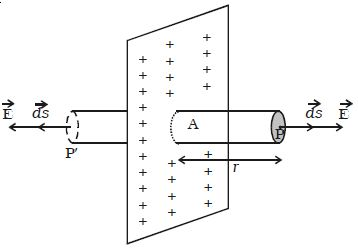Physics

# Application of Gauss’s Law: Electric Field due to an Infinite Charged Plane Sheet

Electric field due to an infinite charged plane sheet (Application of Gauss’s Law):

Consider an infinite plane sheet of charge with surface charge density σ. Let P be a point at a distance r from the sheet (Figure) and E be the electric field at P.

Consider a Gaussian surface in the form of the cylinder of cross− sectional area A and length 2r perpendicular to the sheet of charge.Fig: Infinite plane sheet

By symmetry, the electric field is at right angles to the end caps and away from the plane. Its magnitude is the same at P and at the other cap at P′.

Therefore, the total flux through the closed surface is given by

φ = [ϐ E.ds]p + [ϐ E.ds]p1

so, E A + E A = 2 E A

If σ is the charge per unit area in the plane sheet, then the net positive charge q within the Gaussian surface is, q = σA

Using Gauss’s law, 2 EA = σA/ε0

then, E = σ/2ε0